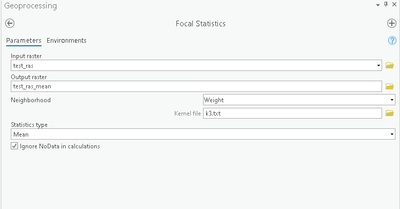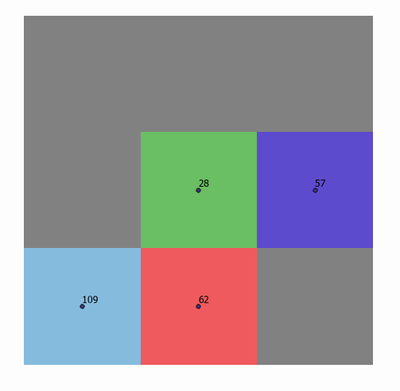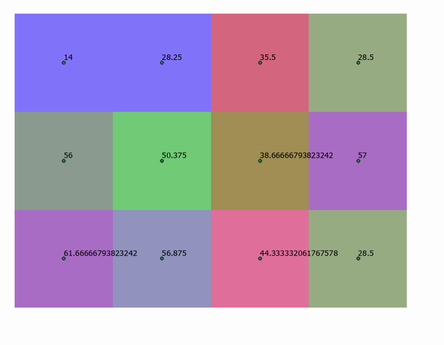# Weighted average using NbrWeight kernel file?

312
3
10-11-2021 12:46 PMNew Contributor III

Hi,

I am trying to calculate a weighted mean statistic using a neighborhood defined by a kernel file:My kernel file defines a 3x3 cell neighborhood, with the following weights:

 0.5 1 0.5 1 1 1 0.5 1 0.5

For illustration purposes, I'll use the following 3x3 raster as an example.  The point labels show the values of the raster cells. Grey cells indicate no data. My target cell for this example is the center green cell, with a value of 28.When I run the tool,  the weighted mean is calculated as:

weighted sum / # of  data cells in neighborhood

For the green target cell: 201.5/4 = 50.375

Output shown below:I want it to be calculated as:

weighted sum / sum of weights of data cells in neighborhood

For the green target cell this should would be 201.5/3.5 =57.57143

How do I calculate this weighted average??

Thank you!

Tags (5)
1 Solution

Accepted SolutionsbyMVP Esteemed Contributor

interesting.

have you tried running the focal sum only,

produce a raster which is classed a nodata or 1 based on the presence of data in your input raster (the inverse of IsNull or a Con statement would do)

If you run a focal sum using your kernel on that raster and you should get your 3.5 value

After that, take your initial focal sum raster and divide it by your focal sum 1's raster

... sort of retired...
3 RepliesbyMVP Esteemed Contributor

interesting.

have you tried running the focal sum only,

produce a raster which is classed a nodata or 1 based on the presence of data in your input raster (the inverse of IsNull or a Con statement would do)

If you run a focal sum using your kernel on that raster and you should get your 3.5 value

After that, take your initial focal sum raster and divide it by your focal sum 1's raster

... sort of retired...New Contributor III

Thanks Dan! This will work. I appreciate the help!byMVP Esteemed Contributor

no problem... an interesting question

... sort of retired...